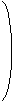# Quaternion Julia Fractals

Written by Paul Bourke
July 2001

What happened on the 3rd February 2004, the birthday of Gaston Julia?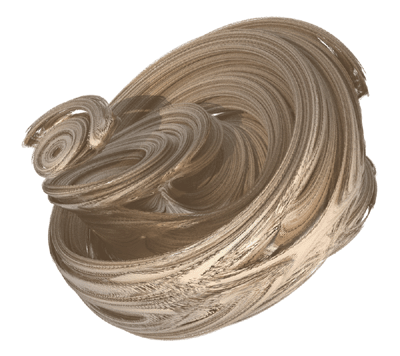Introduction

Quaternion Julia fractals are created by the same principle as the more traditional Julia set except that it uses 4 dimensional complex numbers instead of 2 dimensional complex numbers. A 2D complex number is written as z = r + a i where i2 = -1. A quaternion has two more complex components and might be written as q = r + a i + b j + c k where r, a, b, and c are real numbers. There are slightly more complicated relationships between i, j, and k

 i2 = j2 = k2 = -1 i j = k j k = i k i = j j i = -k k j = -i i k = -j

For more details on quaternion maths (how to add and multiple) see the reference given in the header above.

To generate a quaternion fractal a function is iterated zn+1 = f(zn) and if it tends to infinity then it is outside the Julia set, if it is bounded then it is inside the set. Non linear functions are by far the more interesting, in what follows we use the simplest non linear function zn+1 = zn2 + c where c and z are both quaternions. z0 is the point in quaternion space being considered and c is a constant that identifies the particular quaternion. Another function that is often used is a cubic, namely zn+1 = zn3 + c.

Just as with 2D traditional Julia fractals which are either connected or not depending on the constant c, the same applies to quaternion Julia sets. Some examples of the range of Julia set shapes is given in the column on the right. The disconnected sets aren't represented because they are hard to visualise in 2D, they really require a stereoscopic 3D display and/or projection system. The images created here were rendered using custom software distributed on N separate machines. The goal was to create interactive quaternion sets, at the time of writing 16 machines could render a typical set at about 1 frame every 2 seconds.

Practical matters

The fundamental issue is whether the series "escapes" or not. This cannot always be determined without generating the series to an infinite length, something undesirable in practice. There are two criteria that if they are met allow one to make a decision in a finite time. The first is to assume that if the modulus (length) of a term in the series is larger than some value then the series will escape. This is typically taken to be 4. Another criteria is to limit the number of iterations and if the series hasn't escaped by then, then the point is considered to be inside the set. This maximum number of iterations is often as low as 50, the higher it is the longer the fractal takes to create but the more accurate it is.

Another approximation is the precision at which the 4D quaternion space is sampled. One approach is to relate the pixel resolution of the image to quaternion space and sample one or two times per pixel. Too coarse a sampling risks missing parts of the fractal, a very fine sampling results in a very long compute time. Since these are fractal objects there are good reasons to sample them very finely, this raises interesting questions when one tries to antialias rendering of quaternions by supersampling. Because these are fractal in nature, instead of supersampling generating less noisy surface it can generate more noisy surfaces since there is variation at infinitely small scales.

Dimension reduction

Since it is rather hard to draw 4 dimensional objects one needs a way of rendering 4D quaternion fractals on a 2D screen. The approach used here is to intersect the 4D solid with a plane, in essence this makes one of the quaternion components dependent on the other three. To get a feel for the true nature of the quaternion fractal one needs create a whole series of slices along an axis perpendicular to the slice plane. This is the same as what one does when drawing contour lines to visualise a landscape, each contour represents a slice of the landscape by a plane perpendicular to the vertical axis. By "stacking" the contours together we gain an appreciation of the surface. Unfortunately in the case of a 4D object we need to "stack" 3D solid objects along a 4th axis which is a little more difficult for our limited 3D visual system.
The following shows 6 slices moving the cutting plane along one of the quaternion axes from the origin in steps of 0.1, c = (-0.08,0.0,-0.8,-0.03).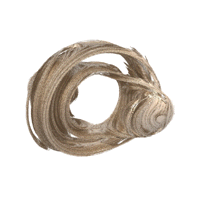Smoothly changing the slice plane results in very attractive animation sequences.

PovRay

If you wish to experiment with quaternion Julia fractals without writing your own code then there is perhaps no better way than by using PovRay, a raytracing rendering program that contains quaternion Julia fractals as a primitive. As a starting point this PovRay scene file may be helpful.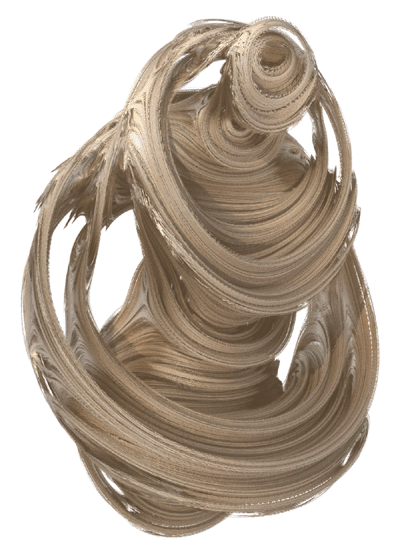c = (-1,0.2,0,0)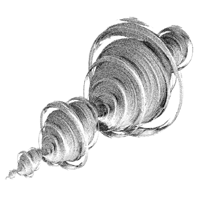c = (-0.291,-0.399,0.339,0.437)c = (-0.2,0.4,-0.4,-0.4)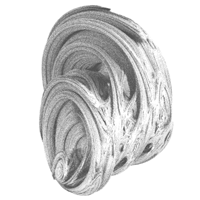c = (-0.213,-0.0410,-0.563,-0.560)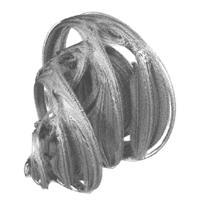c = (-0.2,0.6,0.2,0.2)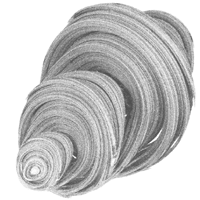c = (-0.162,0.163,0.560,-0.599)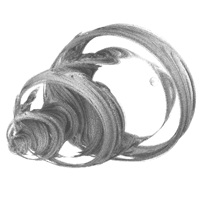c = (-0.2,0.8,0,0)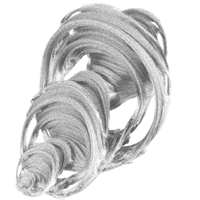c = (-0.445,0.339,-0.0889,-0.562)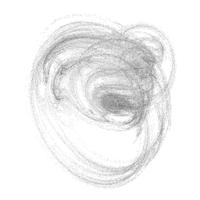c = (0.185,0.478,0.125,-0.392)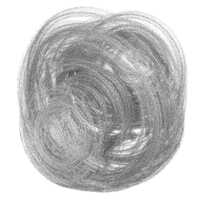c = (-0.450,-0.447,0.181,0.306)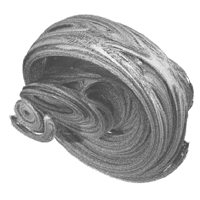c = (-0.218,-0.113,-0.181,-0.496)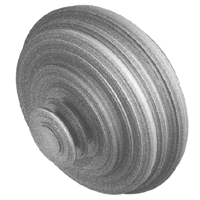c = (-0.137,-0.630,-0.475,-0.046)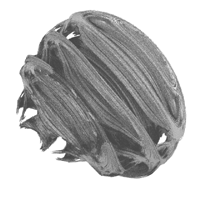c = (-0.125,-0.256,0.847,0.0895)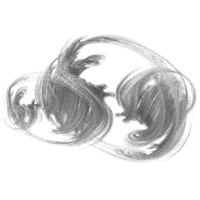# Quaternion Maths

Written by Paul Bourke
June 2001

Most students of algebra are familiar with complex numbers, that is, numbers that have two components called real and imaginary that can often be interpreted as 2 dimensions. A complex number is normally written as a + ib where i2 = -1 and a and b are two real values quantities. This idea can be extended to higher dimensions but it turns out that 4 components have useful properties. These are called quaternions and are attributed to Sir William Rowan Hamilton who published a major analysis in 1844 called "On a Species of Imaginary Quantities Connected with a Theory of Quaternions" in the Proceedings of the Royal Irish Academ (2, pp 424-434)

In this discussion we will write a quaternion "Q" as

Q = r + a i + b j + c k

Where "r" might be considered the real component and "a", "b", "c" real valued. The 4 vector (r,a,b,c) might be considered to be a vector in the 4D quaternion space.

When performing operations on complex numbers whenever one encounters i2 then one knows that is equal to the simpler -1. There are similar but slightly more complicated relationships between i,j,k in quaternion space. They are as follows:

 i2 = j2 = k2 = -1 i j = k j k = i k i = j j i = -k k j = -i i k = -j

Note that the order in which i,j,k appears in an expression is important. Also note that there is no linear relationship between i,j,k.

Addition (or subtraction) of two quaternions Q1 = r1 + a1 i + b1 j + c1 k and Q2 = r2 + a2 i + b2 j + c2 k is performed as follows.

Q1 + Q2 = r1+r2 + (a1+a2) i + (b1+b2) j + (c1 + c2) k
Conjugate

The conjugate of Q = Q* = r - a i - b j - c k.

Multiplication

Multiplication of two quaternions is somewhat involved but follows directly from the relationships above.

 Q1 Q2 = [ r1 r2 - a1 a2 - b1 b2 - c1 c2 ] + [ r1 a2 + a1 r2 + b1 c2 - c1 b2 ] i + [ r1 b2 + b1 r2 + c1 a2 - a1 c2 ] j + [ r1 c2 + c1 r2 + a1 b2 - b1 a2 ] k

Note that quaternion multiplication is not commutative, that is, Q1 Q2 is NOT the same as Q2 Q1

Length (modulus)

The length (magnitude) of a quaternion is the familiar coordinate length in 4 dimensional space.

|Q| = sqrt( Q Q* )

where Q* is the conjugate (see later) which expands to

|Q| = sqrt(r2 + a2 + b2 + c2)

and

|Q1 Q2| = |Q1| |Q2|

Inverse

The inverse of a quaternion Q-1 such that Q Q-1 = 1 is given by

 r - a i - b j - c k Q-1 = ------------------------ |Q|2

The inverse of a normalised quaternion is simply the conjugate, otherwise the magnitude of the inverse is 1/|Q|. So the above expression normalises the quaternion and then scales by 1/|Q|.

Division

Division of Q1 by Q2 is as follows

 Q1 Q1 (2 r2 - Q2) -------- = ------------------- Q2 |Q2|2
Exponential

If m = sqrt(a2 + b2 + c2) and v is the unit vector (a,b,c) / m then the exponential of the quaternion Q is

exp(Q) = exp(r) [ cos(m), v sin(m) ]
Polar Coordinates

The equivalent to polar coordinates in quaternion space are

r = |Q| cos(theta1)
a = |Q| sin(theta1) cos(theta2)
b = |Q| sin(theta1) sin(theta2) cos(theta3)
c = |Q| sin(theta1) sin(theta2) sin(theta3)

theta1 is known as the amplitude of the quaternion, theta2 and theta3 are the latitude (or co-latitude) and longitude respectively. The representative point of a quaternion is the normalised vector (a,b,c), that is, where (a,b,c) intersects the unit sphere centered at the origin.

Rotation of a vector about another vector

To rotate a 3D vector "p" by angle theta about a (unit) axis "r" one forms the quaternion

Q1 = (0,px,py,pz)

and the rotation quaternion

Q2 = (cos(theta/2), rx sin(theta/2), ry sin(theta/2), rz sin(theta/2)).

The rotated vector is the last three components of the quaternion

Q3 = Q2 Q1 Q2*

It is easy to see that rotation in the opposite direction (-theta) can be achieved by reversing the order of the multiplication.

Q3 = Q2* Q1 Q2

Note also that the quaternion Q2 is of unit magnitude, and needs to be in order to be a valid rotation.

Converting a quaternion to a matrix

Given a quaternion rotation the corresponding 3x3 rotation matrix M is given by

M =1 - 2 b2 - 2 c2 2 a b - 2 r c 2 a c + 2 r b 2 a b + 2 r c 1 - 2 a2 - 2 c2 2 b c - 2 r c 2 a c - 2 r b 2 b c + 2 r c 1 - 2 a2 - 2 b2